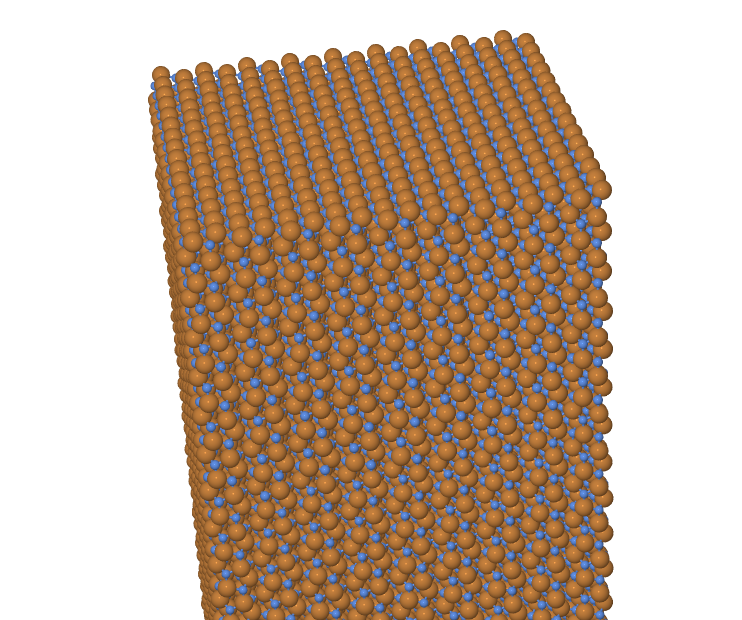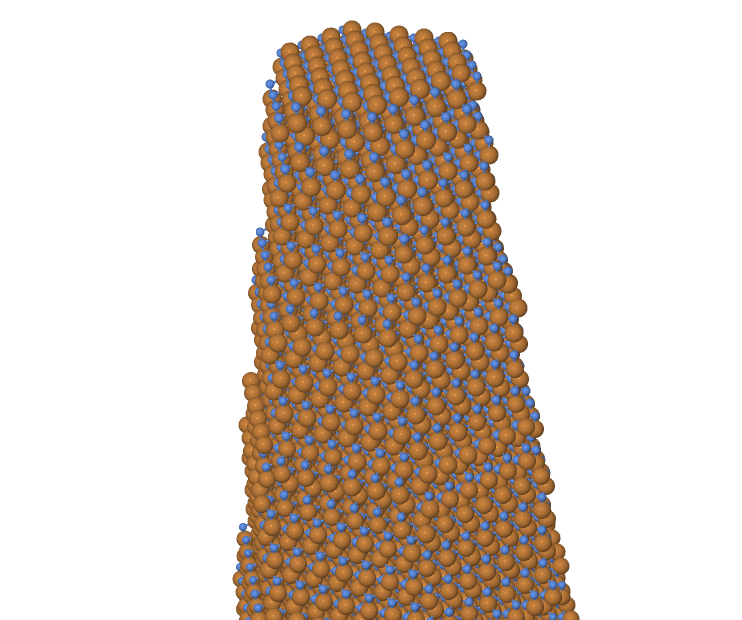Sculpting

Suppose we have a crystal in the form of a rectangular block, given by a list of the absolute atomic coordinates with one atom per line

Ga   -9.278018850000   -9.642000000000   -7.870137870000
N    -7.422415050000   -9.642000000000   -8.518637230000
.
.
.

looking like that:What we really want, though, is a hexagonal column which tapers down from the bottom to the top — just like a column of Doric order. How can we sculpt this column out of the block we have? Where do we get the digital chisel we need? A colleague of mine solved this quest in the most elegant fashion: with an awk one-liner.

awk '{x=sqrt($2^2);y=sqrt($3^2);d=x/2+sqrt(3)/2*y;z=$4;cutoff='$radius1'*('$zmax'-z)/'$zrange'+'$radius2'*(z-('$zmin'))/'\$zrange'};d&lt;=cutoff &amp;&amp; x&lt;cutoff' block.dat &gt; column.dat

Voilà: